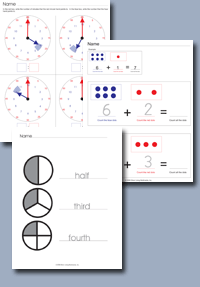﻿ Common Core Standards - MathAdaptive Worksheets

Helping Every Child to Succeed

toll-free (888) 777-0876
fax (888) 777-0875

## Understand addition as putting together and adding to, and understand subtraction as taking apart and taking from.

• K.OA.1. Represent addition and subtraction with objects, fingers, mental images, drawings1, sounds (e.g., claps), acting out situations, verbal explanations, expressions, or equations.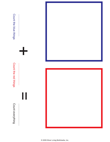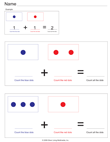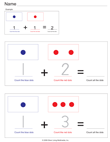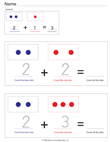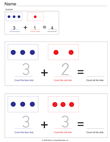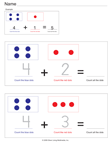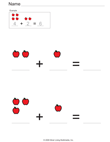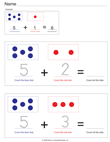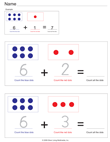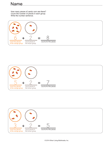Subtraction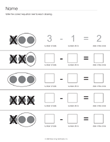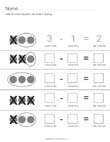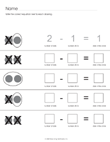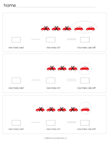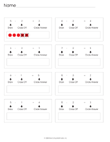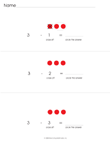• K.OA.2. Solve addition and subtraction word problems, and add and subtract within 10, e.g., by using objects or drawings to represent the problem.

• K.OA.3. Decompose numbers less than or equal to 10 into pairs in more than one way, e.g., by using objects or drawings, and record each decomposition by a drawing or equation (e.g., 5 = 2 + 3 and 5 = 4 + 1).

• K.OA.4. For any number from 1 to 9, find the number that makes 10 when added to the given number, e.g., by using objects or drawings, and record the answer with a drawing or equation.

• K.OA.5. Fluently add and subtract within 5.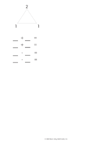Math Families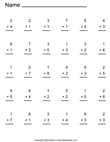Use this math generator to create simple addition worksheets.